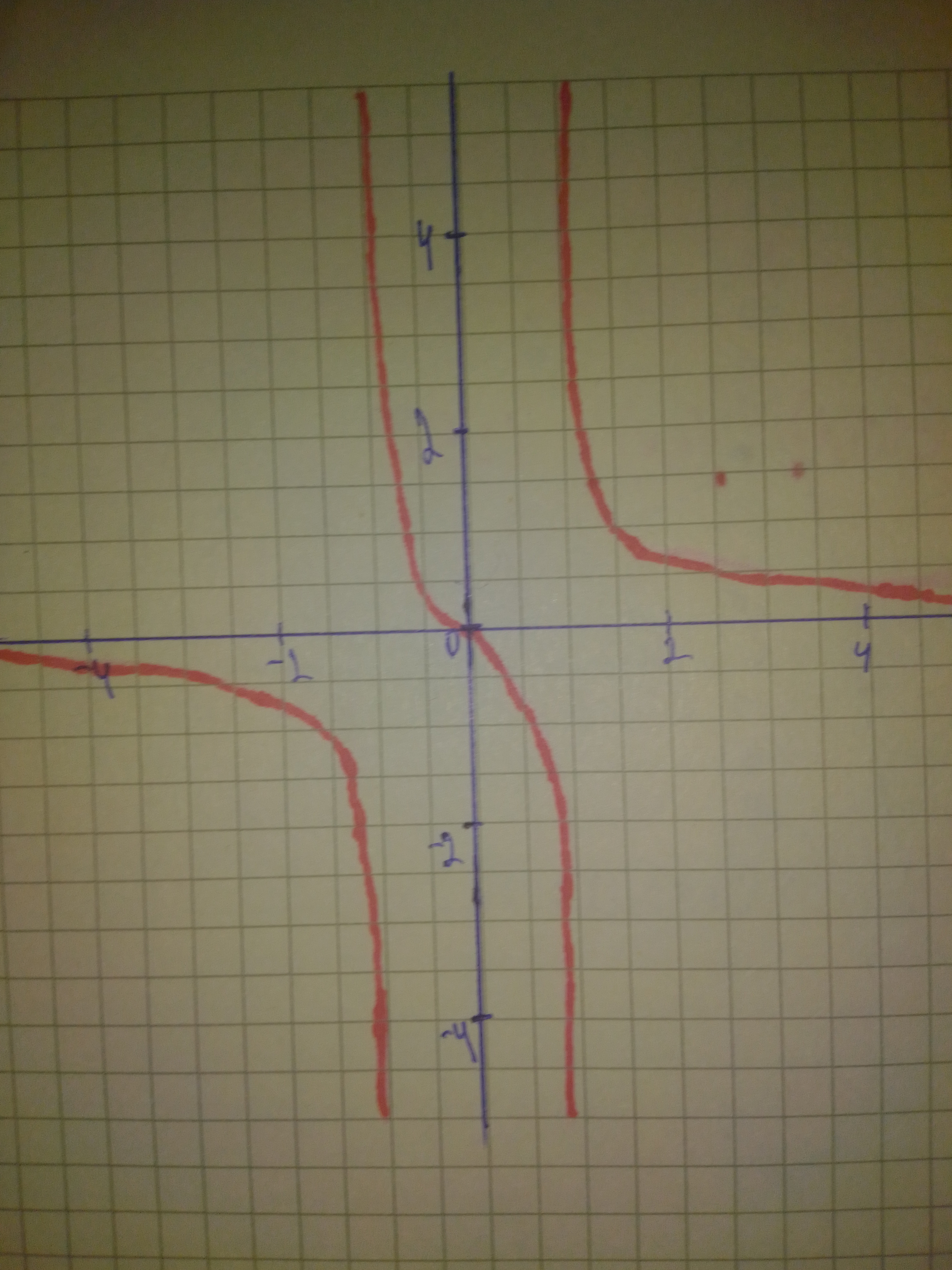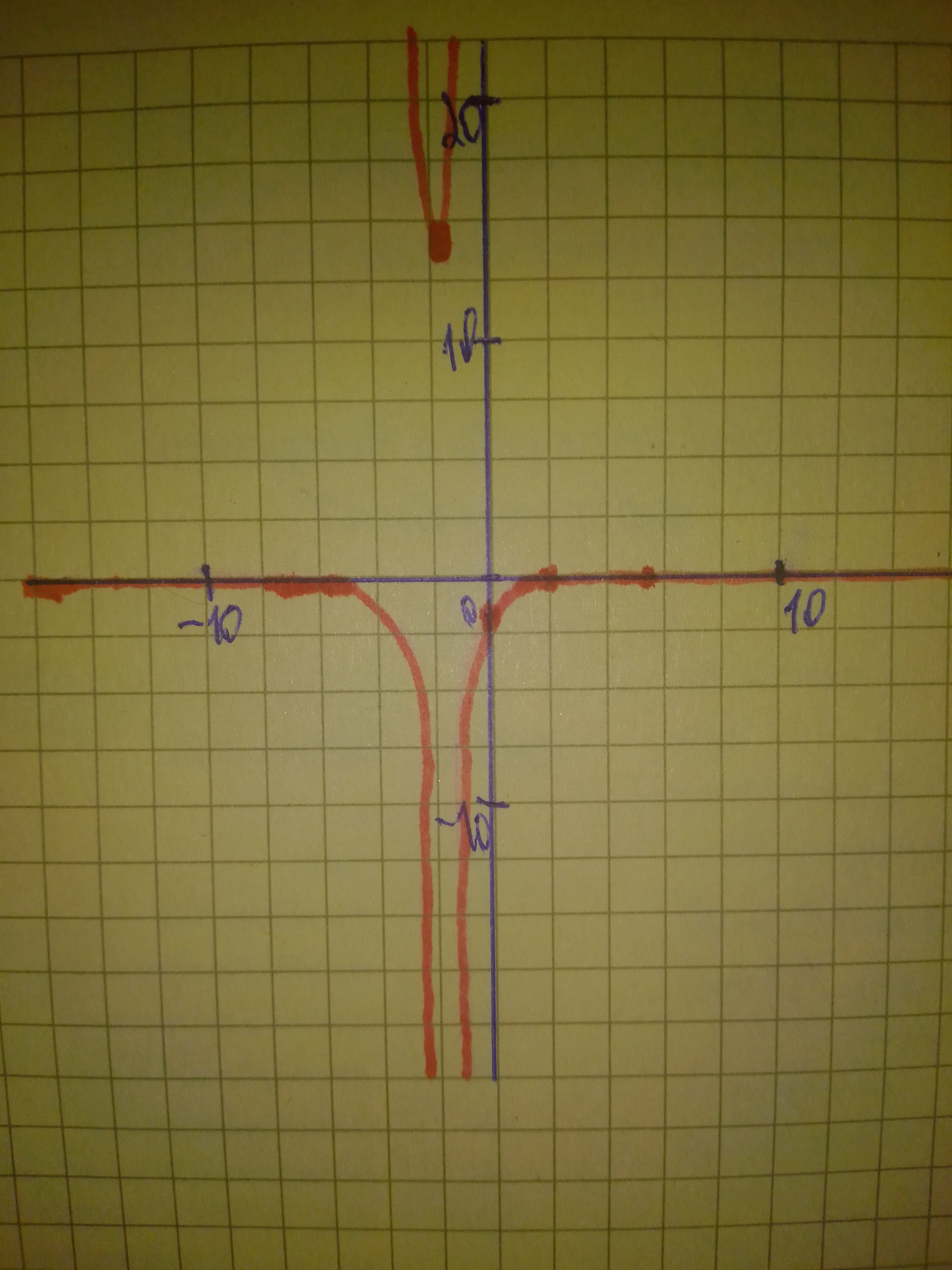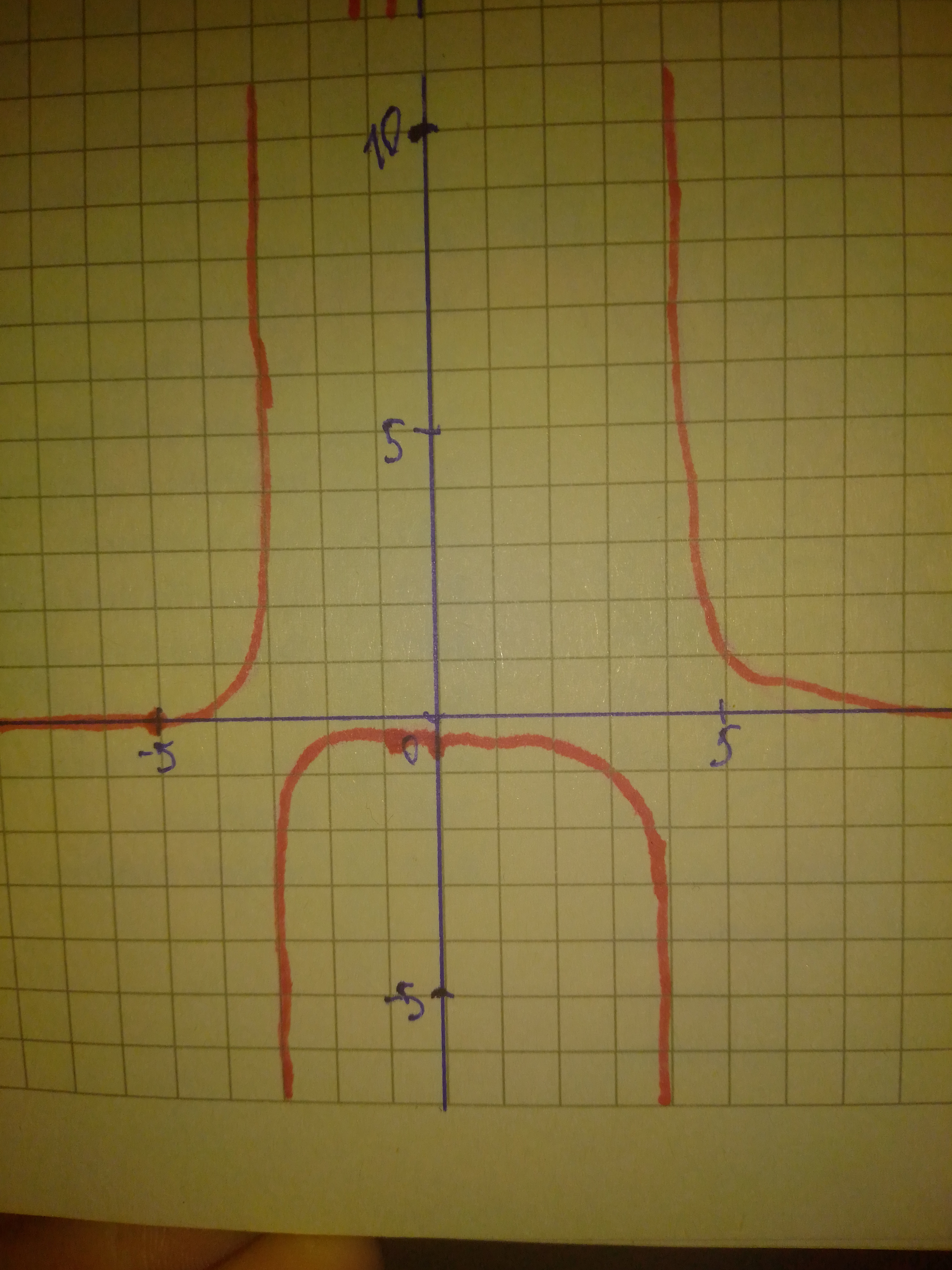Rational functions can have any polynomial in the numerator and denominator. Analyse the key features of each function and sketch its graph. Describe the common features of the graphs. a) f(x)=frac{x}{x^{2}-1} b)g(x)=frac{x-2}{x^{2}+3x+2} c)h(x)=frac{x+5}{x^{2}-x-12}Bevan Mcdonald 2020-12-03 Answered

Rational functions can have any polynomial in the numerator and denominator. Analyse the key features of each function and sketch its graph. Describe the common features of the graphs.

$$\displaystyle{a}{)}{f{{\left({x}\right)}}}={\frac{{{x}}}{{{x}^{{{2}}}-{1}}}}\$$

$${b}{)}{g{{\left({x}\right)}}}={\frac{{{x}-{2}}}{{{x}^{{{2}}}+{3}{x}+{2}}}}\$$

$${c}{)}{h}{\left({x}\right)}={\frac{{{x}+{5}}}{{{x}^{{{2}}}-{x}-{12}}}}$$

• Questions are typically answered in as fast as 30 minutes

Solve your problem for the price of one coffee

• Math expert for every subject
• Pay only if we can solve itjlo2niT

Step 1
a. Function: $$\displaystyle{f{{\left({x}\right)}}}={\frac{{{x}}}{{{x}^{{{2}}}-{1}}}}$$
Step 2 For vertical asymptote, equate the expression in the denominator with 0 and solve for x, therefore: $$\displaystyle{x}^{{{2}}}-{1}={0}$$
Solve for x: $$\displaystyle{x}^{{{2}}}={1}$$
Apply square root on both sides of the equation: $$\displaystyle{x}=\sqrt{{{1}}}=\pm{1}$$
Step 3
The equation of vertical asymptote also gives information about the domain of this function, therefore the domain of $$\displaystyle{f{{\left({x}\right)}}}\ {i}{s}\ {\left(-\infty,-{1}\right)}\cup{\left(-{1},{1}\right)}\cup{\left({1},\infty\right)}$$
Step 4
SInce the degree of the expression in the denominator is 2, greater than the degree of the expression in the numerator, horizontal asymptote of this function is $$\displaystyle{y}={0}$$
Step 5
The range of this function $$\displaystyle{f{{\left({x}\right)}}}\ {i}{s}\ {\left(-\infty,\infty\right)}.$$ Step 6
Graph of $$\displaystyle{f{{\left({x}\right)}}}={\frac{{{x}}}{{{x}^{{{2}}}-{1}}}}$$Step 7
b. Function: $$\displaystyle{g{{\left({x}\right)}}}={\frac{{{x}-{2}}}{{{x}^{{{2}}}+{3}{x}+{2}}}}$$
Step 8
For vertical asymptote, equate the expression in the denominator with 0 and solve for x, therefore: $$\displaystyle{x}^{{{2}}}+{3}{x}+{2}={0}$$
Factor using ac method: $$\displaystyle{x}^{{{2}}}+{x}+{2}{x}+{2}={0}$$
Factor: $$\displaystyle{\left({x}+{2}\right)}{\left({x}+{1}\right)}={0}$$
Solve for x: $$\displaystyle{x}=-{2}$$
And:$$\displaystyle{x}=-{1}$$
The equation of vertical asymptotes also gives information about the domain of this function, therefore the domain of $$\displaystyle{g{{\left({x}\right)}}}\ {i}{s}\ {\left(-\infty,-{2}\right)}\cup{\left(-{2},-{1}\right)}\cup{\left(-{1},\infty\right)}.$$
Step 9
Since the degree of the expression in the denominator is 2, greater than the degree of the expression in the numerator, horizontal asymptote of this function is $$\displaystyle{y}={0}$$
Step 10
The range of this function $$g(x)\ is\ (-\infty, 0) \cup (13,928, \infty)$$ (from graph)
Step 11
Graph of $$\displaystyle{g{{\left({x}\right)}}}={\frac{{{x}-{2}}}{{{x}^{{{2}}}+{3}{x}+{2}}}}$$Step 12
c.Function: $$\displaystyle{h}{\left({x}\right)}={\frac{{{x}+{5}}}{{{x}^{{{2}}}-{x}-{12}}}}$$
Step 13
For vertical asymptote, equate the expression in the denominator with 0 and solve for x, therefore: $$\displaystyle{x}^{{{2}}}-{x}-{12}={0}$$
Factor using ac method: $$\displaystyle{x}^{{{2}}}-{4}{x}+{3}{x}-{12}={0}$$
Factor: $$\displaystyle{\left({x}-{4}\right)}{\left({x}+{3}\right)}={0}$$
Solve for x: $$\displaystyle{x}=-{3}$$
And: $$\displaystyle{x}={4}$$
Step 14
The equation of vertical asymptote also gives information about the domain of this function, therefore the domain of $$\displaystyle{h}{\left({x}\right)}\ {i}{s}\ {\left(-\infty,-{3}\right)}\cup\ {\left(-{3},{4}\right)}\cup{\left({4},\infty\right)}.$$
Step 15
SInce the degree of the expression in the denominator is 2, greater than the degree of the expression in the numerator, horizontal asymptote of this function is $$\displaystyle{y}={0}$$.
Step 16
The range of this function $$h(x)\ is\ (-\infty, -0.398) \cup (-0.398, \infty)$$ (from graph).
Step 17
$$\displaystyle{h}{\left({x}\right)}={\frac{{{x}+{5}}}{{{x}^{{{2}}}-{x}-{12}}}}$$